# Plots of geodesics in Kerr spacetime¶

## Computation with kerrgeodesic_gw¶

This Jupyter/SageMath notebook is relative to the lectures Geometry and physics of black holes.

It requires SageMath (version $\geq$ 8.2), with the package kerrgeodesic_gw (version $\geq$ 0.3). To install the latter, simply run

sage -pip install kerrgeodesic_gw

in a terminal.

In :
version()

Out:
'SageMath version 9.2, Release Date: 2020-10-24'

First, we set up the notebook to use LaTeX-formatted display:

In :
%display latex


and we ask for CPU demanding computations to be performed in parallel on 8 processes:

In :
Parallelism().set(nproc=8)


A Kerr black bole is entirely defined by two parameters $(m, a)$, where $m$ is the black hole mass and $a$ is the black hole angular momentum divided by $m$. In this notebook, we shall set $m=1$ and we denote the angular momentum parameter $a$ by the symbolic variable a, using a0 for a specific numerical value:

In :
a = var('a')
a0 = 0.998


The spacetime object is created as an instance of the class KerrBH:

In :
from kerrgeodesic_gw import KerrBH
M = KerrBH(a)
print(M)

Kerr spacetime M


The Boyer-Lindquist coordinate $r$ of the event horizon:

In :
rH = M.event_horizon_radius()
rH

Out:
In :
rH0 = rH.subs({a: a0})
rH0

Out:

The method boyer_lindquist_coordinates() returns the chart of Boyer-Lindquist coordinates BL and allows the user to instanciate the Python variables (t, r, th, ph) to the coordinates $(t,r,\theta,\phi)$:

In :
BL.<t, r, th, ph> = M.boyer_lindquist_coordinates()
BL

Out:

The metric tensor is naturally returned by the method metric():

In :
g = M.metric()
g.display()

Out:

## Bound timelike geodesic¶

We set $\mu=1$ and pick some values of $E$, $L$ and $Q$, with $E<1$ to ensure that we are dealing with a bound geodesic:

In :
mu = 1
E = 0.9
#L = 1.9
L = 2.
Q = 1.3

In :
r = var('r')
R(r) = ((E^2 - 1)*r^4 + 2*r^3 + (a0^2*(E^2 - 1) - Q - L^2)*r^2
+ 2*(Q + (L - a0*E)^2)*r - a0^2*Q)

In :
graph = plot(-R(r), (r, -1.5, 7.5), thickness=1.5,
axes_labels=[r'$r/m$', r'$-\mathcal{R}(r)/m^4$'])
graph

Out: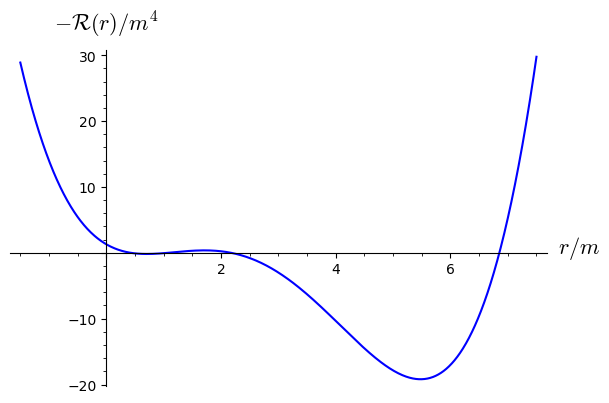In :
rp = find_root(R(r), 1.5, 4)
rp

Out:
In :
ra = find_root(R(r), 5, 10)
ra

Out:
In :
graph += line([(rp, 0), (ra, 0)], thickness=2.5, color='red') \
+ text(r'$r_{\rm p}$', (1.05*rp, -2.5), color='red', fontsize=16) \
+ text(r'$r_{\rm a}$', (1.02*ra, -2.5), color='red', fontsize=16)
graph += line([(rH0, -20), (rH0, 30)], thickness=2, color='black')
graph

Out: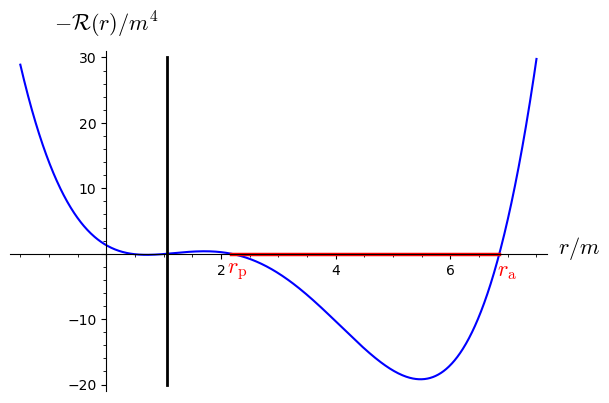In :
zoom = plot(-R(r), (r, -0.1, 2.5), thickness=1.5,
axes_labels=[r'$r/m$', r'$-\mathcal{R}(r)/m^4$'])
zoom += line([(rp, 0), (2.5, 0)], thickness=2.5, color='red') \
+ text(r'$r_{\rm p}$', (1.01*rp, 0.2), color='red', fontsize=12)
zoom += line([(rH0, -0.8), (rH0, 1.8)], thickness=2, color='black')
zoom

Out: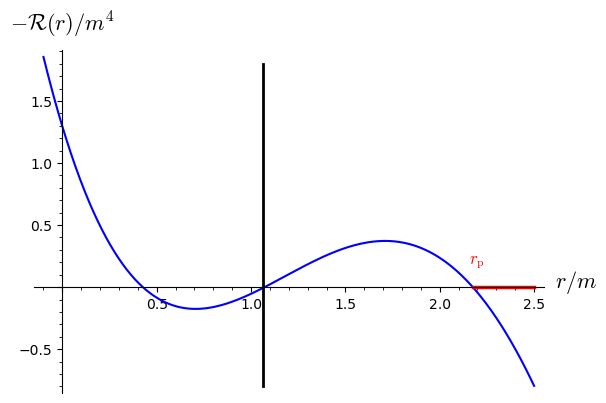In :
graph = graph.inset(zoom, (0.7, 0.7, 0.3, 0.3), fontsize=8)
graph

Out: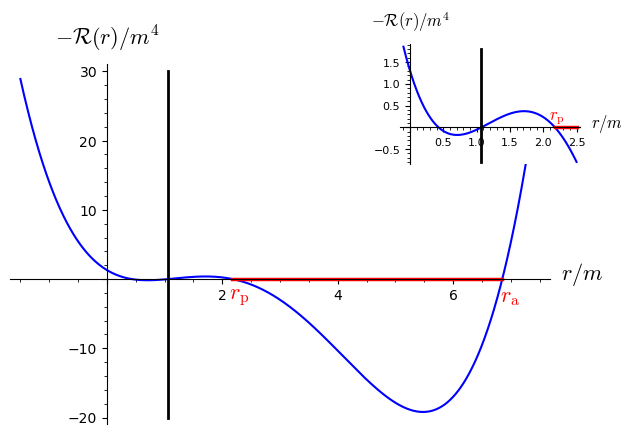In :
graph.save("gek_R_potential.pdf")


Let us choose the initial point $P$ for the geodesic:

In :
P = M.point((0, (rp + ra)/2, pi/2, 0), name='P')
print(P)

Point P on the Kerr spacetime M


A geodesic is constructed by providing the range $[\lambda_{\rm min},\lambda_{\rm max}]$ of the affine parameter $\lambda$, the initial point and either

• (i) the Boyer-Lindquist components $(p^t_0, p^r_0, p^\theta_0, p^\phi_0)$ of the initial 4-momentum vector $p_0 = \left. \frac{\mathrm{d}x}{\mathrm{d}\lambda}\right| _{\lambda_{\rm min}}$,
• (ii) the four integral of motions $(\mu, E, L, Q)$
• or (iii) some of the components of $p_0$ along with with some integrals of motion. We shall also specify some numerical value for the Kerr spin parameter $a$.

Here, we choose $\lambda\in[0, 600\, m]$, the option (ii) and $a=0.998 \,m$, where $m$ in the black hole mass::

In :
lmax = 600 # lambda_max

In :
Li = M.geodesic([0, lmax], P, mu=mu, E=E, L=L, Q=Q, a_num=0.998,
name='Li', latex_name=r'\mathcal{L}', verbose=True)

Initial tangent vector:

The curve was correctly set.
Parameters appearing in the differential system defining the curve are [a].

In :
print(Li)

Geodesic Li of the Kerr spacetime M


The numerical integration of the geodesic equation is performed via integrate(), by providing the integration step $\delta\lambda$ in units of $m$:

In :
Li.integrate(step=0.005)


We can then plot the geodesic:

In :
Li.plot(plot_points=2000, thickness=1.5)

Out:

Actually, many options can be passed to the method plot(). For instance to a get a 3D spacetime diagram:

In :
Li.plot(coordinates='txy', thickness=2)

Out:

or to get the trace of the geodesic in the $(x,y)$ plane:

In :
graph = Li.plot(coordinates='xy', plot_points=2000, thickness=1.5,
axes_labels=[r'$x/m$', r'$y/m$'])
graph

Out: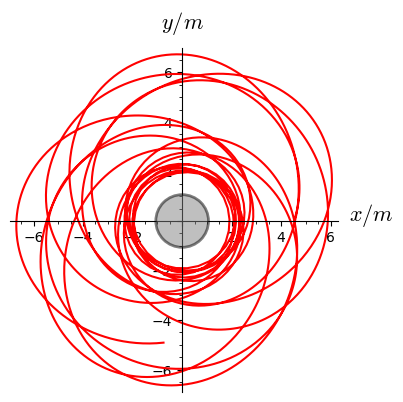In :
graph.save("gek_timelike_xy.pdf")


or else to get the trace in the $(x,z)$ plane:

In :
graph = Li.plot(coordinates='xz', plot_points=2000, thickness=1.5,
axes_labels=[r'$x/m$', r'$z/m$'])
graph

Out: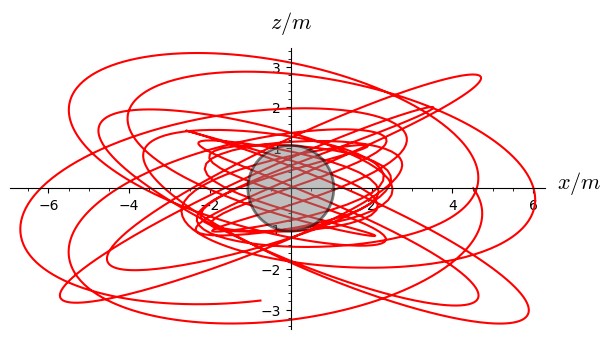In :
graph.save("gek_timelike_xz.pdf")

In :
th = var('th', latex_name=r'\theta')
V(th) = cos(th)^2 * (a0^2*(1 - E^2) + L^2/sin(th)^2)
V(th)

Out:
In :
graph = plot(V(th), (th, 0.7, pi-0.7), axes_labels=[r'$\theta$', r'$V(\theta)$'])
graph += line([(0, Q), (pi, Q)], color='red')
graph

Out: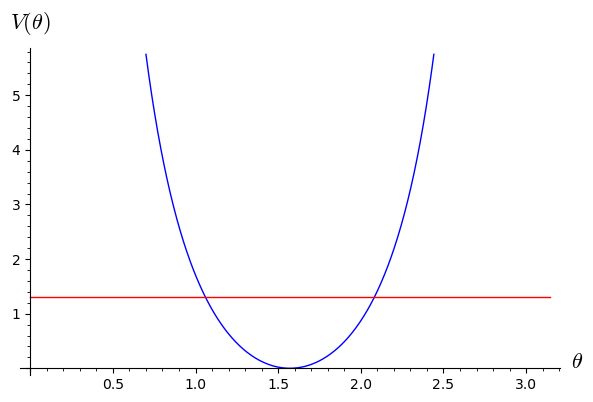In :
th_min = find_root(V(th) - Q, 0.5, pi/2)
th_min

Out:

Analytic formula for $\theta_{\rm min}$:

In :
A2 = a0^2*(mu^2 - E^2)
sthm = (A2 - L^2 - Q + sqrt((L^2 + Q - A2)^2 + 4*L^2*A2))/(2*A2)
th_min0 = arcsin(sqrt(sthm))
th_min0

Out:
In :
th_min - th_min0

Out:
In :
th_min_deg = n(th_min/pi*180)
th_min_deg

Out:
In :
th_inc_deg = 90 - th_min_deg
th_inc_deg

Out:
In :
p_K = 2*ra*rp/(ra + rp)
p_K

Out:
In :
e_K = (ra - rp)/(ra + rp)
e_K

Out:

Let us check that the values of $\mu$, $E$, $L$ and $Q$ evaluated at $\lambda=300 \, m$ are equal to those at $\lambda=0$ up to the numerical accuracy of the integration scheme:

In :
Li.check_integrals_of_motion(lmax)

Out:
 quantity value initial value diff. relative diff. $\mu$ $0.9999976785567484$ $1.0$ $-2.321 \times 10^{-6}$ $-2.321 \times 10^{-6}$ $E$ $0.899985179453538$ $0.900000000000000$ $-0.00001482$ $-0.00001647$ $L$ $1.99982956873133$ $2.00000000000000$ $-0.0001704$ $-0.00008522$ $Q$ $1.29980808952398$ $1.30000000000000$ $-0.0001919$ $-0.0001476$

Decreasing the integration step leads to smaller errors:

In :
Li.integrate(step=0.001)
Li.check_integrals_of_motion(lmax)

Out:
 quantity value initial value diff. relative diff. $\mu$ $0.999999536576446$ $1.0$ $-4.634 \times 10^{-7}$ $-4.634 \times 10^{-7}$ $E$ $0.899997039643679$ $0.900000000000000$ $-2.960 \times 10^{-6}$ $-3.289 \times 10^{-6}$ $L$ $1.99996601125880$ $2.00000000000000$ $-0.00003399$ $-0.00001699$ $Q$ $1.29996145780904$ $1.30000000000000$ $-0.00003854$ $-0.00002965$

## Ingoing null geodesic with negative angular momentum¶

We choose a ingoing null geodesic in the equatorial plane with $L = -6 E < 0$, starting at the point of Boyer-Lindquist coordinates $(t,r,\theta,\phi) = (0, 12, \pi/2, 0)$:

In :
lmax = 13.07
Li = M.geodesic([0, lmax], M((0,12,pi/2,0)), mu=0, E=1, L=-6, Q=0,
r_increase=False, a_num=a0,
name='Li', latex_name=r'\mathcal{L}', verbose=True)

Initial tangent vector:

The curve was correctly set.
Parameters appearing in the differential system defining the curve are [a].

In :
Li.integrate(step=0.00002)

In :
graph = Li.plot(coordinates='xy', color='green', prange=[0, lmax], plot_points=40000,
thickness=1.5, display_tangent=True, scale=0.0001,
color_tangent='green', plot_points_tangent=4,
axes_labels=[r'$x/m$', r'$y/m$'])
graph

Out: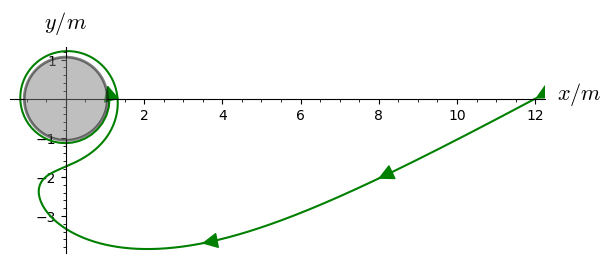In :
graph.save('gek_winding_null.pdf')

In :
Li.check_integrals_of_motion(0.9999*lmax)

Out:
 quantity value initial value diff. relative diff. $\mu$ $0.00444310201139289$ $5.47028152480290 \times 10^{-9}$ $0.004443$ $812200.$ $E$ $1.00000024333053$ $1.00000000000000$ $2.433 \times 10^{-7}$ $2.433 \times 10^{-7}$ $L$ $-5.99999942161639$ $-6.00000000000000$ $5.784 \times 10^{-7}$ $-9.640 \times 10^{-8}$ $Q$ $1.31244009464357 \times 10^{-31}$ $0$ $1.312 \times 10^{-31}$ -

## Ingoing time geodesic with zero angular momentum¶

In :
lmax = 26.41
Li = M.geodesic([0, lmax], M((0,15,pi/2,0)), mu=1, E=1, L=0, Q=0,
r_increase=False, a_num=a0,
name='Li', latex_name=r'\mathcal{L}', verbose=True)

Initial tangent vector:

The curve was correctly set.
Parameters appearing in the differential system defining the curve are [a].

In :
Li.integrate(step=0.0002)

In :
graph = Li.plot(coordinates='xy', color='red', prange=[0, lmax], plot_points=40000,
thickness=1.5, display_tangent=True, scale=0.0001,
color_tangent='red', plot_points_tangent=6,
axes_labels=[r'$x/m$', r'$y/m$'])
show(graph, ymin=-2, ymax=3)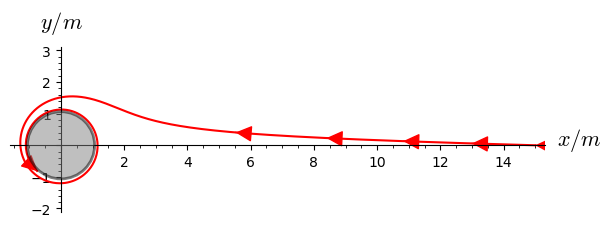In :
graph.save('gek_frame_dragging.pdf')

In :
Li.check_integrals_of_motion(0.9999*lmax)

Out:
 quantity value initial value diff. relative diff. $\mu$ $1.00001380801330$ $1.00000000000000$ $0.00001381$ $0.00001381$ $E$ $1.00000064318726$ $1.00000000000000$ $6.432 \times 10^{-7}$ $6.432 \times 10^{-7}$ $L$ $1.45776391491381 \times 10^{-6}$ $2.77555756156289 \times 10^{-17}$ $1.458 \times 10^{-6}$ $5.252 \times 10^{10}$ $Q$ $9.83266149777550 \times 10^{-38}$ $0$ $9.833 \times 10^{-38}$ -
In [ ]: